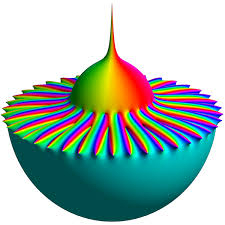# ABS, ARG, CONJ, RECIPROCAL

Let z=-√2-√2i where i2 = -1. Find |z|, arg(z), z* (where * indicates the complex conjugate) and (1/z). Where appropriate, write your answers in the form a + i b,
where both a and b are real numbers.
Indicate the positions of z, z* and (1/z) on an Argand diagram.

Result

a =  2
φ =  0.7854
Φ =  45 °
c = (Correct answer is: -√2+√2i)d = (Correct answer is: -√2/4 + √2/4 i)### Step-by-step explanation:Did you find an error or inaccuracy? Feel free to write us. Thank you!

Tips for related online calculators
Need help calculating sum, simplifying, or multiplying fractions? Try our fraction calculator.
Try our complex numbers calculator.